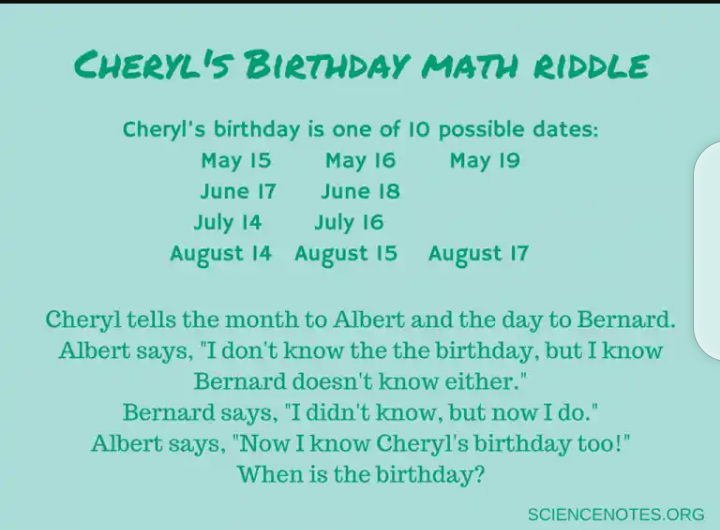Sign in# Cool Math Tricks to Amaze Your Friends

## Cool Math Tricks to Amaze Your friends

Math is like magic, except better! Here is a collection of cool math tricks you can learn to amaze your friends and learn more about how numbers work.

## The 11 Rule

Everyone knows the “10 Rule” where you multiply by 10 simply by adding a 0 to the end of a number, but you might not know the 11 rule. This rule works for any two digit number to multiply it by 11:

For an example, let’s use the number 62

• Separate the two digits in your mind (6 __ 2).
• Add together the two digits of the numbers. (6 + 2 = 8).
• Place this number in the space or hole between the two digits (6 8 2).
• That’s it! 11 x 62 = 682

The only tricky part to remember is that if adding the two digits results in a number greater than 9, then you put the “ones” digit in the space and carry the “tens” digit. For example:

11 x 57 … 5 __ 7 … 5 + 7 = 12

so you put the 2 in the space and add the 1 to the 5, giving you the number 627

11 x 57 = 627

## The 1089 Math Trick

Think of a 3 digit number.

Arrange the digits in descending order.

Reverse the order and subtract it from the number in step 2.

Reverse the order of the answer.

Add it to the result from step 3. You get 1089!

For example, let’s say I chose 423.

Arrange in descending order: 432

Reverse the order and subtract it from the previous number: 234… 432 – 234 = 198

Reverse the order: 891

Add the numbers together: 198 + 891 = 1089

For example, the number is 245.

Arranged in descending order it’s 542.

Reversing the order is 245.

542 – 245 = 297

Reversed, it’s 792

792 + 297 = 1089

## The Answer Is 5

Think of a number.

Double it.

Divide it by 2.

Subtract your original number.

## The Answer Is 2

Think of a number.

Multiply it by 3.

Divide the new number by 3.

Subtract your original number from this one.

The answer is 2.

For example, the number 2345.

Multiplied by 3, it’s 7035.

Adding 6 gives 7041.

Dividing by 3 gives 2347.

Subtracting the original: 2347 – 2347 = 2.

## How To Solve Cheryl’s Birthday Math Riddle“Cheryl’s Birthday” is more of a math riddle or logic puzzle that is solved using the process of elimination or deductive reasoning. Albert can’t know the birthday because he only has the month and all months have multiple dates, but he has enough information to know Bernard does not have the date. If Cheryl had told Bernard 19 or 18, then he would know the whole birthday because there is only one month with each number. This rules out May 19 and June 18.

Since Albert knows Bernard doesn’t know, Albert must have been told July or August, as this rules out any possibility of Bernard being told 18 or 19. This excludes any dates in May or June.

When Bernard says he did not know the answer, but now he does, this means Bernard has the one remaining unique number in the list. If Bernard had a 14, he wouldn’t know whether it was in July or August. If he had a 15 or a 17, he wouldn’t know which date in August was correct. Thus Cheryl’s birthday must be July 16!

If you want to get really tricky, you can rephrase the riddle to say Bernard starts out saying he doesn’t know when the birthday is, with Albert replying he doesn’t know either. If Bernard then says he didn’t know, but now he does, and Albert replies he now knows too, then the answer is August 17. Can you see why?

## Drop your comment below for more tricks

Content created and supplied by: Frinodcomedy2 (via Opera News )